#Function Repository Resource:

# TagSystem

Compute the evolution of a tag system

Contributed by: Stephen Wolfram
 ResourceFunction["TagSystem"][{n,rule},init,t] generates a list representing the evolution of the tag system with the specified rule, where n elements are removed from the beginning of the sequence starting from initial condition init for t steps.

## Details and Options

The rules for a tag system are given as a list of elements of the form {i1rhs1,i2rhs2,} where in are integers and the rhsn are the replacing integers or an empty list.

## Examples

### Basic Examples

Five steps in the evolution of a tag system:

 In:=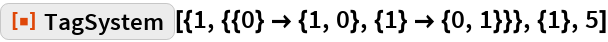Out=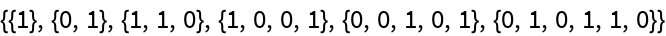Plot the evolution of a tag system:

 In:=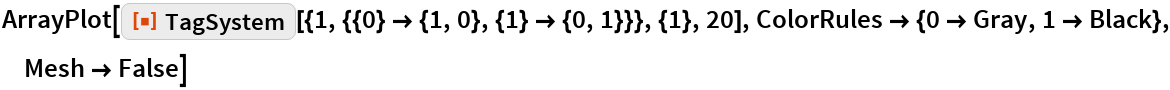Out=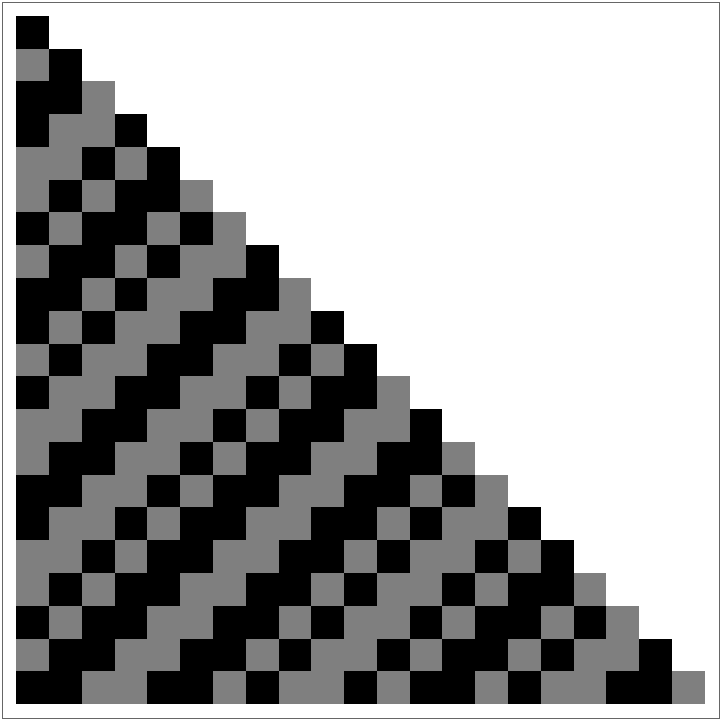Rules from A New Kind of Science, page 93:

 In:=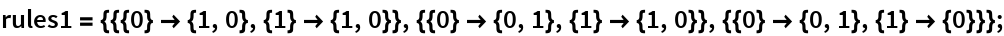In:=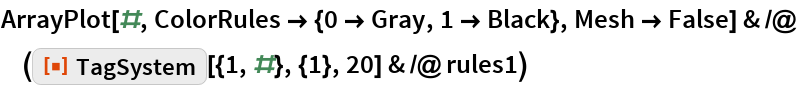Out=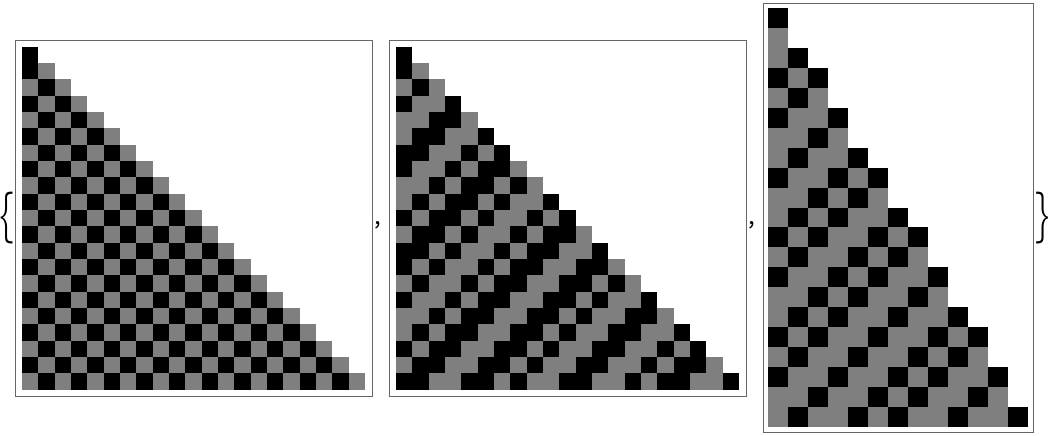Rules from A New Kind of Science, page 94:

 In:=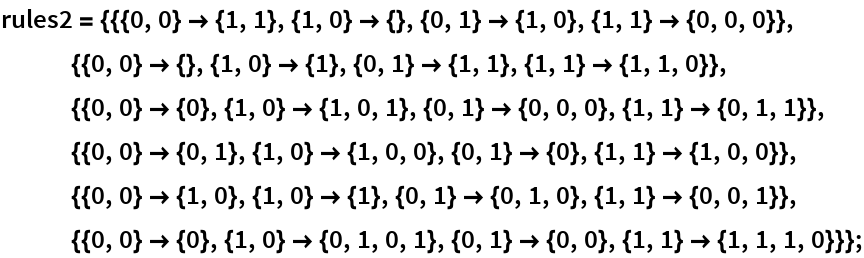In:=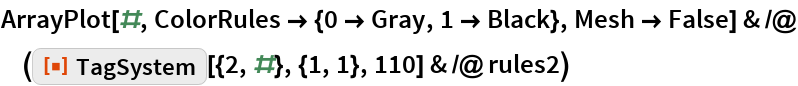Out=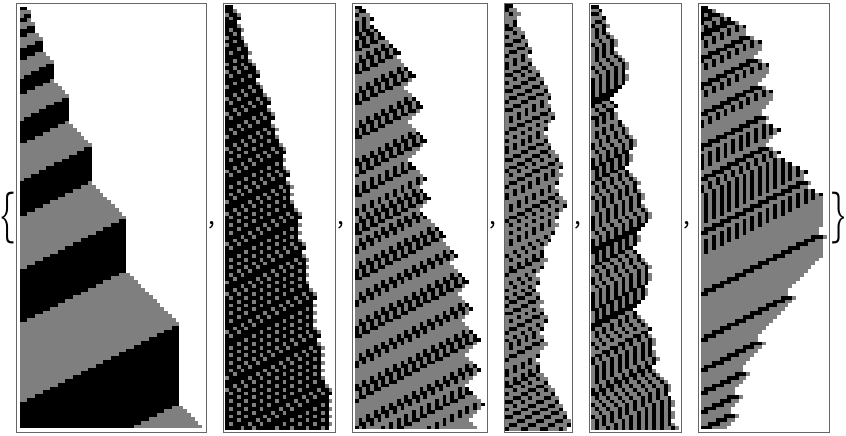Total lengths for rules from A New Kind of Science, page 94:

 In:=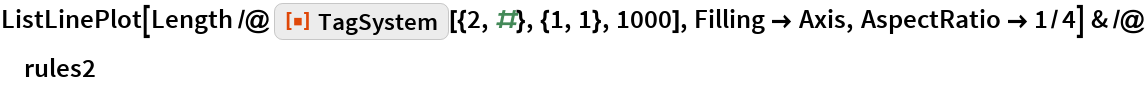Out=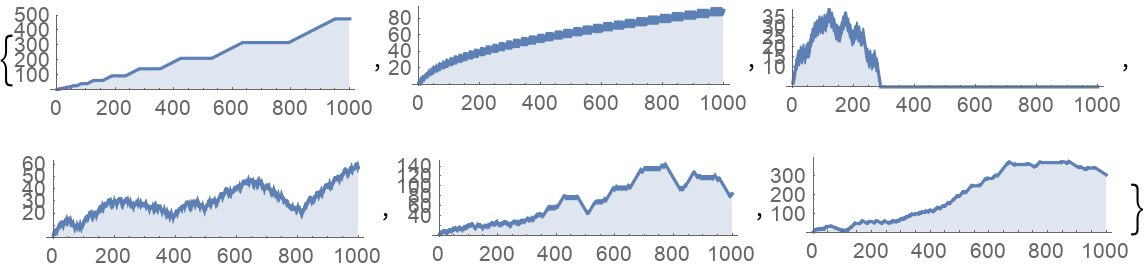## Requirements

Wolfram Language 11.3 (March 2018) or above